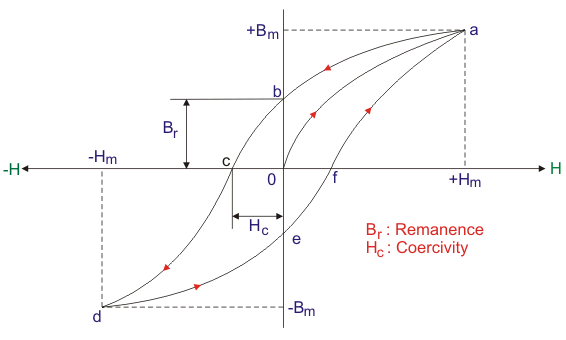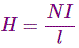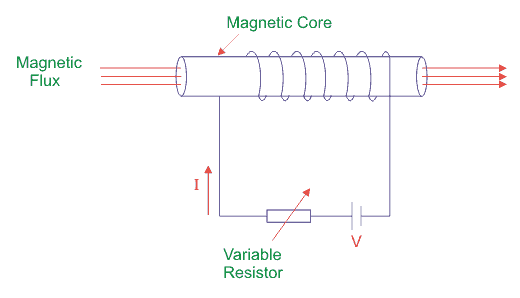# Hysteresis Loop: What is it (And What is its Significance)?

Contents

## What is a Hysteresis Loop?

A hysteresis loop (also known as a hysteresis curve) is a four-quadrant graph that shows the relationship between the induced magnetic flux density B and the magnetizing force H. It is often referred to as the B-H loop. From hysteresis loops, we can determine a number of magnetic properties about a material. Such as the retentivity, residual magnetism (or residual flux), coercive force, permeability, and the reluctance.

An example hysteresis loop is shown below.To understand a hysteresis loop, let’s suppose we take a magnetic material to use as a core around which insulated wire is wound.

The coils are connected to a DC supply through a variable resistor to vary the current “I”. We know that current I is directly proportional to the value of magnetizing force (H) asWhere N = number of turns of coil and l is the effective length of the coil. The magnetic flux density of this core is B which is directly proportional to magnetizing force H.Now, we should be familiar with some important terms related to a hysteresis loop.

### Definition of Hysteresis

Hysteresis of a magnetic material is a property by virtue of which the flux density (B) of this material lags behind the magnetizing force (H).

### Definition of Coercive Force

Coercive force is defined as the negative value of magnetizing force (-H) that reduces residual flux density of a material to zero.

### Residual Flux Density

Residual flux density is the certain value of magnetic flux per unit area that remains in the magnetic material without presence of magnetizing force (i.e. H = 0).

### Definition of Retentivity

It is defined as the degree to which a magnetic material gains its magnetism after magnetizing force (H) is reduced to zero.
Now, let us proceed step by step to make a clear idea about hysteresis loop.• Step 1:
When supply current I = 0, so no existence of flux density (B) and magnetizing force (H). The corresponding point is ‘O’ in the graph above.
• Step 2:
When current is increased from zero value to a certain value, magnetizing force (H) and flux density (B) both are set up and increased following the path o – a.
• Step 3:
For a certain value of current, flux density (B) becomes maximum (Bmax). The point indicates the magnetic saturation or maximum flux density of this core material. All element of core material get aligned perfectly. Hence Hmax is marked on H axis. So no change of value of B with further increment of H occurs beyond point ‘a’.
• Step 4:
When the value of current is decreased from its value of magnetic flux saturation, H is decreased along with decrement of B not following the previous path rather following the curve a – b.
• Step 5:
The point ‘b’ indicates H = 0 for I = 0 with a certain value of B. This lagging of B behind H is called hysteresis. The point ‘b’ explains that after removing magnetizing force (H), magnetism property with little value remains in this magnetic material it is known as residual magnetism (Br). Here o – b is the value of residual flux density due to retentivity of the material.
• Step 6:
If the direction of the current I is reversed, the direction of H also gets reversed. The increment of H in reverse direction following path b – c decreases the value of residual magnetism (Br) that gets zero at point ‘c’ with certain negative value of H. This negative value of H is called coercive force (Hc)
• Step 7:
H is increased more in negative direction further; B gets reverses following path c – d. At point‘d’, again magnetic saturation takes place but in opposite direction with respect to previous case. At point‘d’, B and H get maximum values in reverse direction, i.e. (-Bm and -Hm).
• Step 8:
If we decrease the value of H in this direction, again B decreases following the path de. At point ‘e’, H gets zero-valued, but B is with finite value. The point ‘e’ stands for residual magnetism (-Br) of the magnetic core material in opposite direction with respect to previous case.
• Step 9:
If the direction of H again reversed by reversing the current I, then residual magnetism or residual flux density (-Br) again decreases and gets zero at point ‘f’ following the path e – f. Again further increment of H, the value of B increases from zero to its maximum value or saturation level at point a following path f – a.

The path a – b – c – d – e – f – a forms hysteresis loop.
[NB: The shape and the size of the hysteresis loop depend on the nature of the material chosen]

## Significance of Hysteresis Loops

The main advantages of hysteresis loops are given below.

1. Smaller hysteresis loop area symbolizes less hysteresis loss.
2. Hysteresis loop provides the value of retentivity and coercivity of a material. Thus the way to choose perfect material to make permanent magnet, core of machines becomes easier.
3. From B-H graph, residual magnetism can be determined and thus choosing of material for electromagnets is easy.

Want To Learn Faster? 🎓
Get electrical articles delivered to your inbox every week.
No credit card required—it’s 100% free.